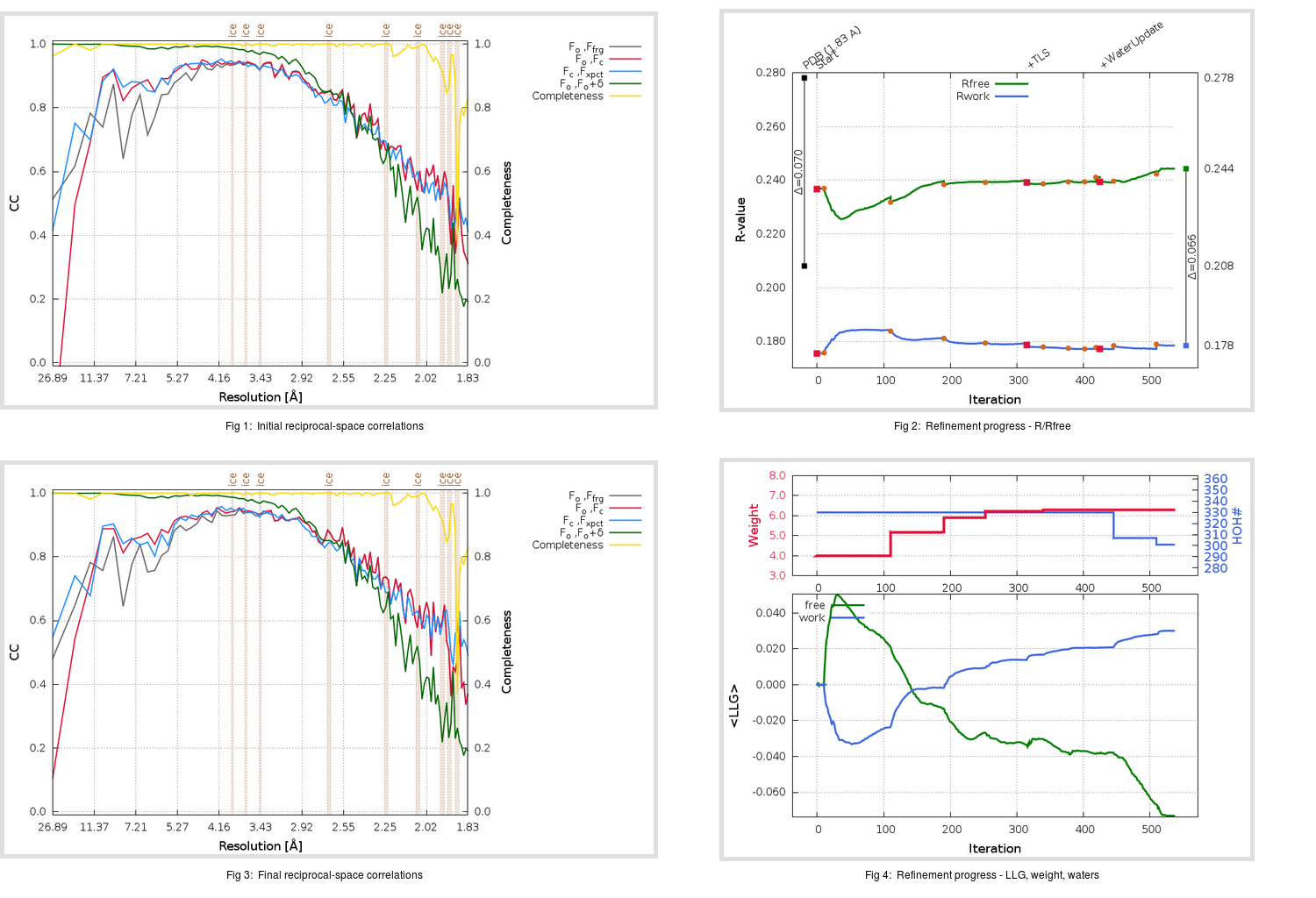Content:

## Deposited

` `
 Date deposited Date data collection Resolution R, Rfree 20200315 20200305 1.83 0.2050 0.2780

Molprobity (CCP4 7.0 version) summary:

```Ramachandran outliers =   0.33 %
favored =  93.05 %
Rotamer outliers      =   2.65 %
C-beta deviations     =     0
Clashscore            =   7.60
RMS(bonds)            =   0.0137
RMS(angles)           =   1.86
MolProbity score      =   2.18
Resolution            =   1.83
R-work                =   0.2050
R-free                =   0.2780
```

```Number of waters      =   330

<B> (all atoms) =   27.74 ( sd =    9.66 ) for       2729 non-hydrogen atoms
<B>   (protein) =   26.73 ( sd =    8.93 ) for       2370 non-hydrogen atoms
<B>     (water) =   34.20 ( sd =   10.52 ) for        330 non-hydrogen atoms
<B>    (others) =   37.61 ( sd =   22.17 ) for         29 non-hydrogen atoms

B min/max       (all non-hydrogen atoms) =   13.26 /   85.75
B min/max   (protein non-hydrogen atoms) =   13.57 /   85.75
B min/max     (water non-hydrogen atoms) =   13.26 /   81.55
B min/max     (other non-hydrogen atoms) =   21.71 /   67.14
```

## BUSTER (re-)refinement

` `

Molprobity (CCP4 7.0 version) summary:

```Ramachandran outliers =   0.33 %
favored =  97.02 %
Rotamer outliers      =   1.14 %
C-beta deviations     =     0
Clashscore            =   3.17
RMS(bonds)            =   0.0116
RMS(angles)           =   1.58
MolProbity score      =   1.32
Resolution            =   1.83
R-work                =   0.1785
R-free                =   0.2443
```

```Number of waters      =   301

<B> (all atoms) =   29.78 ( sd =    8.40 ) for       2700 non-hydrogen atoms
<B>   (protein) =   28.71 ( sd =    7.13 ) for       2370 non-hydrogen atoms
<B>     (water) =   37.10 ( sd =   11.27 ) for        301 non-hydrogen atoms
<B>    (others) =   41.70 ( sd =   22.33 ) for         29 non-hydrogen atoms

B min/max       (all non-hydrogen atoms) =    6.40 /  129.66
B min/max   (protein non-hydrogen atoms) =   17.78 /   61.33
B min/max     (water non-hydrogen atoms) =    6.40 /  129.66
B min/max     (other non-hydrogen atoms) =   26.09 /   61.37
```

Refinement progression:Results:

` `
 File Remark 5RFO_aB_refine.01_03_refine.pdb.gz exact refinement commands are in header 5RFO_aB_refine.01_03_refine.mtz.gz including original deposited data and several re-refinement map coefficients 5RFO_aB_refine.01_03_BUSTER_model.cif.gz including any non-standard compound restraints 5RFO_aB_refine.01_03_BUSTER_refln.cif.gz